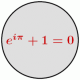## Tail Bounds of the Normal Distribution

The question of bounding the tails of the normal distribution has popped up a couple of times on math.SE lately.  This is an easy-to-prove but useful result, and so it’s worth talking about.

The standard normal probability density function is$f(t) = \frac{1}{\sqrt{2\pi}} e^{-t^2/2}$.  We want to find a bound on$\int_x^{\infty} \frac{1}{\sqrt{2\pi}} e^{-t^2/2} dt$ for$x > 0$.

One simple way, since$\frac{t}{x} \geq 1$, is the following:$\displaystyle \int_x^{\infty} \frac{1}{\sqrt{2\pi}} e^{-t^2/2} dt \leq \int_x^{\infty} \frac{t}{x} \frac{1}{\sqrt{2\pi}} e^{-t^2/2} dt = \frac{e^{-x^2/2}}{x\sqrt{2\pi}}.$

We can get improved bounds, though, by using repeated integration by parts.  Ignoring the constant for now, introduce$\frac{t}{t}$ to the integrand.  Then let$u = \frac{1}{t}$ and$dv = t e^{-t^2/2} dt$.  Thus$du = - \frac{1}{t^2}$ and$v = - e^{-t^2/2} dt$.  We get$\displaystyle \int_x^{\infty} e^{-t^2/2} dt = -\left.\frac{e^{-t^2/2}}{t}\right|_x^{\infty} - \int_x^{\infty} \frac{e^{-t^2/2}}{t^2} dt = \frac{e^{-x^2/2}}{x} - \int_x^{\infty} \frac{e^{-t^2/2}}{t^2} dt.$

Since the integral at the end is negative, we have the same bound as before:$\int_x^{\infty} \frac{1}{\sqrt{2\pi}} e^{-t^2/2} dt \leq \frac{e^{-x^2/2}}{x\sqrt{2\pi}}.$  However, instead of stopping here we could continue to apply integration by parts to the integral on the right-hand side.  For example, the next application (with$u = \frac{1}{t^3}$ and$dv = t e^{-t^2/2} dt$) yields a lower bound:$\displaystyle \int_x^{\infty} \frac{1}{\sqrt{2\pi}} e^{-t^2/2} dt \geq \frac{e^{-x^2/2}}{\sqrt{2\pi}} \left(\frac{1}{x} - \frac{1}{x^3}\right).$

(See, for example, Dilip Sarwaite’s answer here.)  Continuing this process will produce successively better and better upper and lower bounds.

Added (January 23, 2012):  Recently I found this nice technical report, “Bounding Standard Gaussian Tail Probabilities,” by Lutz Dümbgen.  It surveys many known results on bounding the tails of the normal distribution and refines some of them.  I modified one of the derivations in the report to prove an inequality that was asked about on math.SE recently.

This entry was posted in probability, statistics. Bookmark the permalink.

### 6 Responses to Tail Bounds of the Normal Distribution

1.cardinal says:

Hi Mike. Nice to see you’ve started this blog. I always enjoy reading your answers on math.SE. Note that the last display equation in your post has the inequality flipped. The right-hand side is a lower bound. There are some more interesting related results that I’ve been meaning to put into a MO answer for awhile now. I’ll send you a note when (if?) that happens.

•mzspivey says:

Thanks, cardinal! I’ve fixed the mistake you pointed out. And please do let me know when/if you post those related results.

2.Rob Johnson says:

Perhaps it’s more than you are looking for here, but I posted an asymptotic expansion for the tail on math.SE: http://math.stackexchange.com/questions/145087/how-to-calculate-the-integral-in-normal-distribution/177992#177992

•mzspivey says:

Thanks, Rob.

3. Pingback: Gaussian tail bounds – Beth ​'s blog